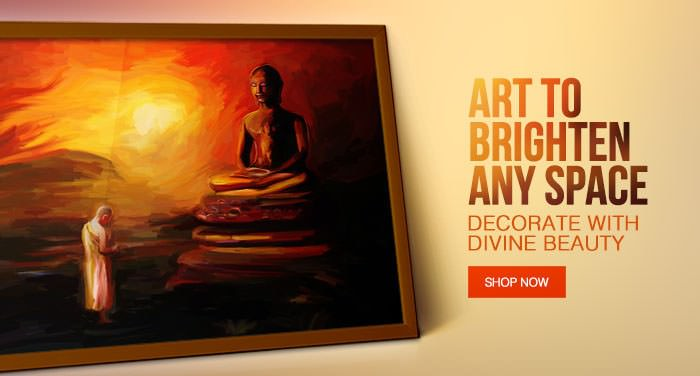Subscribe to RSS Feed

Jain ArtItems 1 to 20 of 25 total

Set Ascending Direction
per page

Grid  List

Page:

Starting at
Rs. 1,700.00

Starting at
Rs. 2,100.00

Starting at
Rs. 1,700.00

Starting at
Rs. 1,700.00

Starting at
Rs. 1,700.00

Starting at
Rs. 2,100.00

Starting at
Rs. 2,100.00

Starting at
Rs. 1,700.00

Starting at
Rs. 2,100.00

Starting at
Rs. 2,100.00

Starting at
Rs. 1,700.00

Starting at
Rs. 1,700.00

Starting at
Rs. 2,100.00

Starting at
Rs. 1,700.00

Starting at
Rs. 1,700.00

Starting at
Rs. 1,700.00

Starting at
Rs. 1,700.00

Starting at
Rs. 2,100.00

Starting at
Rs. 2,100.00
• The blue flame

Starting at
Rs. 1,700.00

Items 1 to 20 of 25 total

Set Ascending Direction
per page

Grid  List

Page: